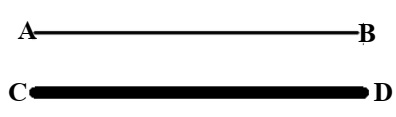ICSE Class 10 Physics 2022 Semester-2 Sample Paper

Class 10 Physics

Maximum marks are 40. Time allowed is one and a half hours. You have to attempt all questions from Section A and any three questions from Section B.

Section A

Question 1

Choose the correct answers to the questions from the given options.

(i) Pendulums A, B, C and D are tied to a flexible string PQ and are at rest. Pendulum C is disturbed. Which of the following statements is true?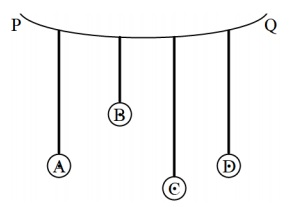(a) Only pendulum C will start vibrating.
(b) Pendulums A, B, and D will also start vibrating but A and D will vibrate with the maximum amplitude.
(c) Pendulums A, B, and D will also start vibrating.
(d) Vibrations of pendulum C are forced vibrations.

(ii) Which of the following is not a characteristic of parallel combination of resistors?

(a) If one resistor is fused, the circuit does not become open.
(b) The total resistance R is given by the formula 1/R = 1/R1 + 1/R2 + 1/R3 + ...
(c) The total resistance becomes less than the least resistor, present in the combination.
(d) The current through each resistor always remains the same.

(iii) Which one of the following statements is correct?

(a) Live wire has zero potential.
(b) Fuse is connected in a neutral wire.
(c) Potential of live and earth wire is always the same.
(d) Earth wire is used to prevent electric shock.

(iv) The diagram below shows a free conductor AB is kept in a magnetic field and is carrying current from A to B. (To avoid confusion complete path of the circuit is not shown) The direction of the force experienced by the conductor will be: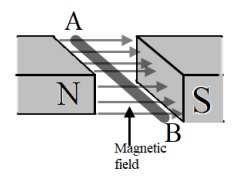(a) Up
(b) Down
(c) Towards N
(d) Towards S

(v) The diagram below shows a magnet moved near a coil along its axis. Which of the diagram shows correct flow of current during this motion?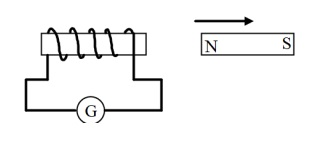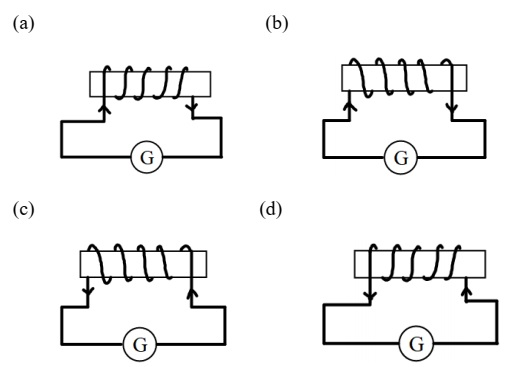(vi) The meaning of the statement ‘Specific heat capacity of water is 4200 Jkg-1K-1’ is:

(a) Water needs 4200 J heat to raise its temperature by 1 kelvin.
(b) To raise the temperature of water 4200 J of heat is absorbed.
(c) 1 kg water absorbs 4200 J heat to increase its temperature by 1 kelvin.
(d) 1 kg Water needs 1 kelvin temperature to absorb 4200 J heat.

(vii) 200 g of ice at 0°C needs _________ heat to melt. [Specific latent heat of ice = 336000 J kg-1]

(a) 6720 J
(b) 67200 J
(c) 672000 J
(d) 67.2 J

(viii) The radiation with maximum penetrating power is:

(a) γ
(b) β
(d) α

(ix) Resonance is:

(a) A forced vibration in which amplitude remains constant.
(b) A forced vibration in which frequency of forced vibration is greater than the free vibrations of the body.
(c) A forced vibration, in which frequency of forced vibration is equal to the free vibrations of the body.
(d) A forced vibration, in which frequency of forced vibration is less than the free vibrations of the body.

(x) The nuclear radiation which gets deflected towards negatively charged plate in an electric field is:

(a) Gamma
(b) Ultraviolet
(c) Beta
(d) Alpha

Section B

Question 2

(i) (a) Calculate the total resistance across AB.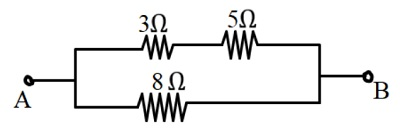(b) If a cell of e.m.f 2.4 V with negligible internal resistance is connected across AB then calculate the current drawn from the cell. 

(ii) (a) Which will absorb more heat, 10 g of ice at 0°C or 10 g of water at 0°C?

(b) For the same mass of ice and ice-cold water, why does ice produce more cooling than ice-cold water? 

(iii) The diagram below shows an insulated copper wire wound around a hollow card board cylindrical tube. Answer the questions that follow: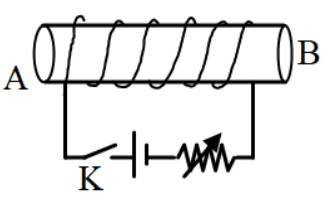1. What are the magnetic poles at A and B when the key K is closed?
2. State two ways to increase the strength of the magnetic field in this coil without changing the coil.
3. If we place a soft iron bar at the centre of the hollow cardboard and replace the DC source by an AC source then will it attract small iron pins toward itself when the current is flowing through the coil?

Question 3

(i) The diagram below shows a cooling curve for 200 g of water. The heat is extracted at the rate of 100 Js-1. Answer the questions that follow: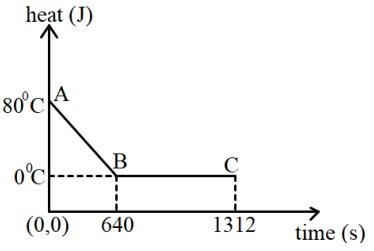1. Calculate specific heat capacity of water.
2. Heat released in the region BC.

(ii) (a) Observe the diagram given below and state whether the bulb will glow or not when we switch on K.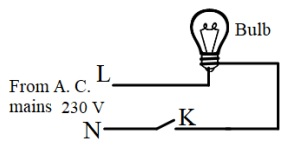(b) Is it safe to handle the bulb when the switch is OFF?

(iii) Two metals A and B have specific heat capacities in the ratio 2:3. If they are supplied same amount of heat then 

1. Which metal piece will show greater rise in temperature given their masses are the same?
2. Which metal piece will have greater mass if the rise in temperature is the same for both metals?
3. If the mass ratio of metal A and metal B is 3:5 then calculate the ratio in which their temperatures rise.
4. If specific heat capacity of metal A is 0.26 Jg-1 °C-1 then calculate the specific heat capacity of metal B.

Question 4

(i) (a) Which one of the following graphs A or B shows free vibrations in vacuum and which one shows free vibrations in a medium?

(b) How did you come to this conclusion.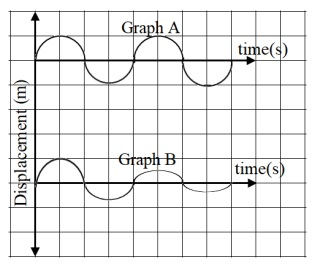(ii) (a) State the Faraday’s laws of electromagnetic induction

(b) Name one electrical device which works on this principle. 

(iii) A nucleus 19482X emits an alpha particle 

1. What will be the atomic number of the daughter nucleus Y?
2. What will be the number of neutrons in the daughter nucleus Y?
3. Write a nuclear reaction showing the emission of this particle.

Question 5

(i) (a) Name the electrical appliance shown in the diagram below.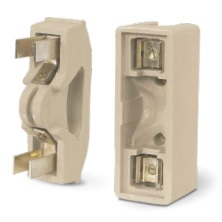(b) Name the material of the wire used in this device.

(c) Name two important characteristics of this wire. 

(ii) (a) Define pitch.

(b) Two wires AB and CD of same length are stretched by same amount. Which wire will produce sound of greater pitch on plucking?